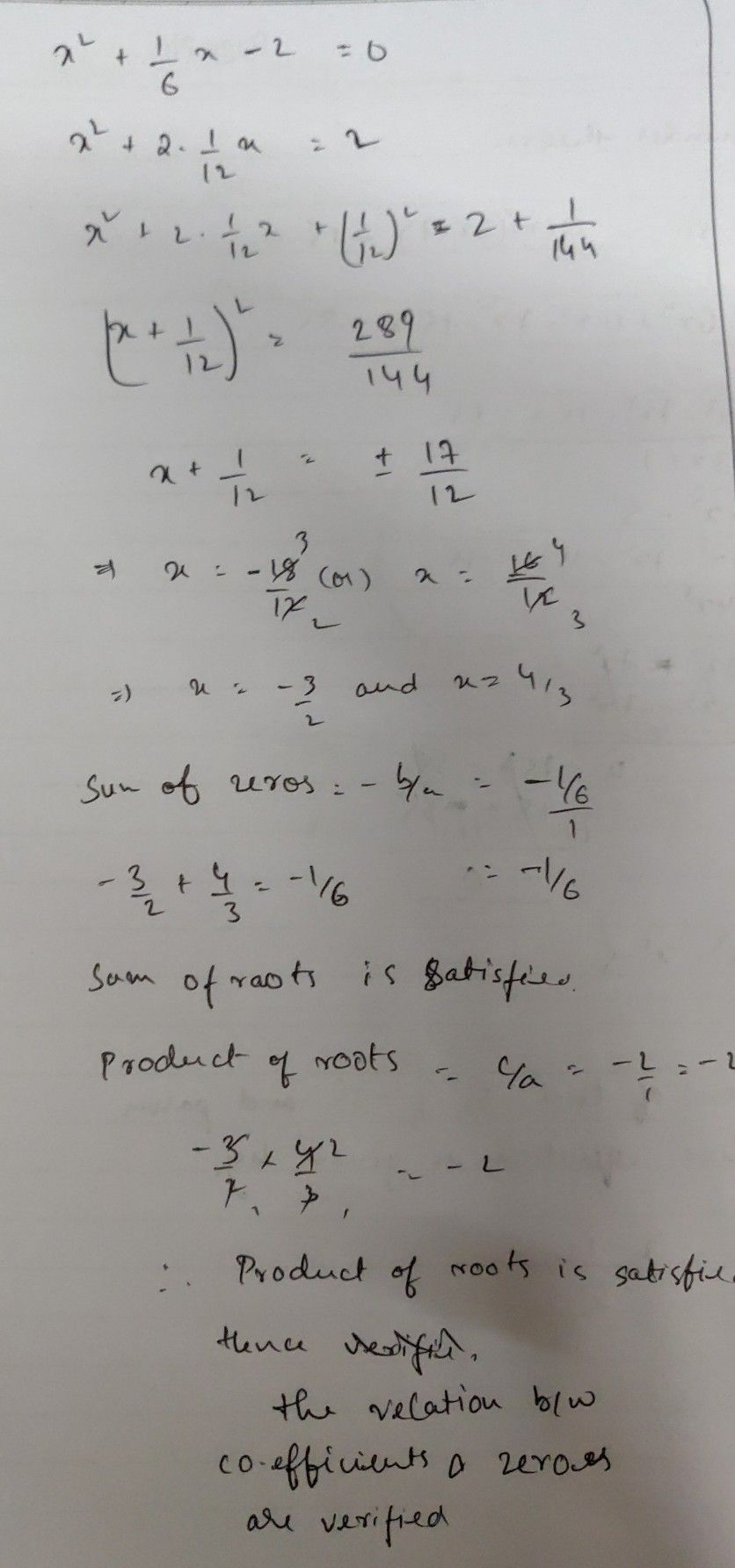Symbol
ProblemFind the zeroes polynomial $1x^{2}$ $\dfrac {1} {6}x$ $22\pi d$ verify the relation between the coefficients and the zeroes of the above polynomial. $1y$ $24$ Find the zeroes of the quadratic polynomial $|x^{2}+7x$ $+10$ and verify the relationship between the zeroes and the coefficients. (NCERT)
1st-6th grade
Algebra
Search count: 105
SolutionQanda teacher - Ajay